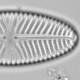## Extracting data from a PDF image

Some scientists archive their data. Some scientists email their data on request. Some editors cajole authors into releasing data to interested parties. And sometimes none of these approaches yields data.

What then? One option is to request data via the scientist’s head-of-department, another is to scrape the data out of published diagrams. Much has been written about how to extract data from raster formats, including stratigraphic diagrams, I only found a little information on how to extract data from vector formats. This is potentially a much more powerful option.

This is how I did it. First I downloaded Luoto and Ojala (2017) and checked that the stratigraphic plot was a vector format by greatly increasing the magnification. This would make the image appear pixelated if it was a raster.The stratigraphic diagram from Luoto and Ojala (2017)

The pdf needs to be converted into a format that can be read into a text editor. This can be done with `qpdf` in the terminal. First I extract the page with the stratigraphic diagram to make further processing easier.

```qpdf 0959683616675940.pdf  --pages 0959683616675940.pdf 4 -- outfile.pdf

qpdf outfile.pdf --stream-data=uncompress  outfile2.pdf
```

Now `outfile2.pdf` can be read into R

```page4 = readLines("luoto/data/outfile2.pdf")

#Find start and end of image
start = grep("/PlacedGraphic", page4)
end = grep("[(Figur)20(e 2.)]TJ", page4, fixed = TRUE)
page4 = page4[start:end]

fig2 = page4[grepl("re\$", page4)] %>%
set_names(c("x", "y", "width", "height", "re"))
```

The bars are, within rounding error, the same height. Various other rectangles in the figure are different heights, so I need to filter the data I want.

```
fig2 = fig2 %>%
filter(between(height, -2.4, -2.3))
```

Now I can plot the data.

```fig2 %>%
ggplot(aes(x = x + width/2, y = y, width = width, height = height, fill = factor(x, levels = sample(unique(x))))) +
geom_tile(show.legend = FALSE)
```The scraped data from the stratigraphic plot

The next step would be to assign taxon names to each unique value of x and scale the widths so they are in percent. When that is done, and the weather data digitised, I can test how well I can reproduce the calibration-in-time transfer function model. The calibration-in-space model will need to wait for data from several other papers to be scraped.1.Kaustubh Thirumalai (@holy_kau) says: